## Mathematics in Careers

The following mathematics investigations for year 10 to 12 students were developed in collaboration with various Industry partners, to showcase how maths is applied in real industry scenarios to solve problems.

The investigations demonstrate the types of thinking and skills that industry require, while applying mathematics that is relevant to the curriculum and real world contexts to bring learning to life.

The activities have been used at MAV’s maths camps to successfully engage students:

• The investigations use various mathematical skills, including data analysis and representation, modelling, problem solving and critical thinking to apply mathematics in real world scenarios.
• Investigations showcase a variety of careers sectors that are underpinned by mathematics.
• Through the activities students will be engaged in exploring various related careers and understanding the importance of mathematics to success in these fields.
• Students and teacher versions of each investigation are available and can be downloaded and used in your classroom.
• Investigations are aimed at year 10 to 12 students.

MAV would like to thank the Australian Centre for Career Education and the Victorian Department of Education and Training for their support in developing these activities.#### Mathematical focus

Body Mass Index (BMI)

Sport Scientist, dietician, personal trainer

• Using formulas to solve problems
• Re-arranging expressions to make a specified variable the subject
• Using authentic data to construct scatter plots, make comparisons and draw conclusionsStudent resources

Teacher notes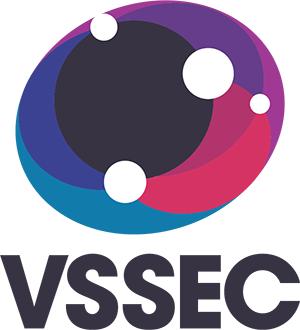Bone Mineral Density (BMD)

Health & Biomedical sciences

• Use appropriate methods to sort, organise, and manipulate data
• Create graphs to show patterns in data
• Explore the connection between algebraic and graphical representations of relations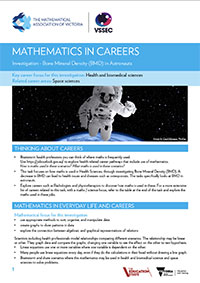Student resources

Teacher notesCell Size

Biologist, zoologist, environmental scientist

• Using formulas to solve problems
• Re-arranging expressions to make a specified variable the subject
• Using authentic situations to apply knowledge and understanding of surface area and volume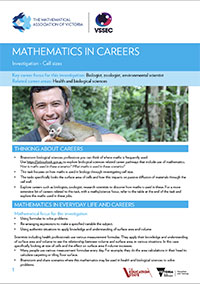Student resources

Teacher notesCollision

Engineering and design

• Solving simple equations arising from formulas
• Re-arranging expressions to make a specified variable the subject
• Calculate speed, time, and distance
• Represent word problems with simple linear equations and solving them to answer questions
• Comparing and analysing data to draw conclusions
• Kinetics (physics)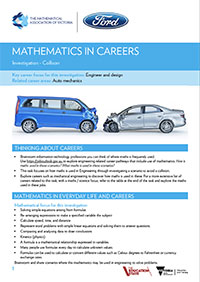Student resource

Teacher notes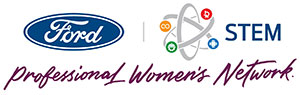Vehicle Safety

Engineering and design

• Represent worded problem with simple linear equations and solve them to answer questions.
• Solving simple equations arising from formulas
• Calculate speed, time, and distance
• Comparing and analysing data to draw conclusionsStudent resource

Teacher notesExploring changes in the cash rate and its effect on the economy

Economics, Commerce

• Represent word problem with simple linear equations and solving them to answer questions
• Solving simple equations arising from formulas
• Re-arranging expressions to make a specified variable the subject
• Associating the solution of simultaneous equations with the coordinates of the intersection of their corresponding graphs.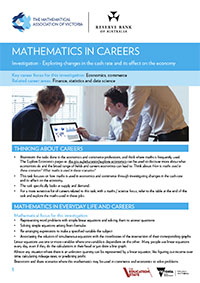Student resource

Teacher notes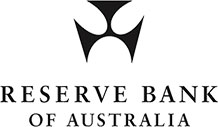Comparing student ability and question difficulty

Educational Research

• Create graphs to show patterns in data
• Explore the connection between algebraic and graphical representations of relations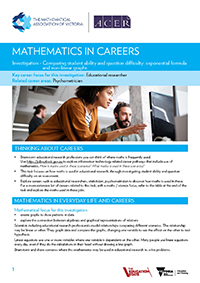Student resource

Teacher notes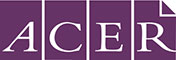Food Industry and coding

Software engineer, coding, information technology

• Using formulas to solve problems
• Using authentic situations to apply knowledge to solve real world problems
• Implement algorithm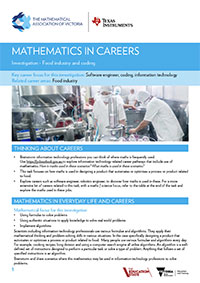Student resource

Teacher notesPanel Cutting

Software engineer, coding, information technology

• Using formulas to solve problems
• Implement algorithms
• Using authentic situations to apply knowledge to solve real world problems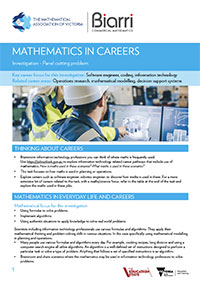Student resource

Teacher notes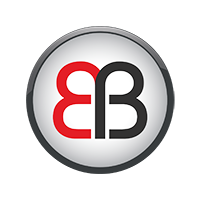Pet Alarm

Software engineer, coding, information technology

• Using formulas to solve problems
• Using authentic situations to apply knowledge to solve real world problems
• Implement algorithmsStudent resource

Teacher notes